## book reviewsFOUNDATIONSADVANCES
ISSN: 2053-2733
Volume 72| Part 1| January 2016| Pages 173-175

The Basics of Crystallography and Diffraction. Fourth Edition. By Christopher Hammond. IUCr Texts on Crystallography, No. 21. IUCr/Oxford Science Publications, 2015. Paperback, Pp. 544. Price GBP 34.99. ISBN 978-0-19-873868-8 .

aResearch with Neutrons and Muons, Paul Scherrer Institut, WHGA/342, Villigen PSI, CH-5232, Switzerland
*Correspondence e-mail: hans.grimmer@psi.ch

The Basics of Crystallography and Diffraction is a textbook written for students at the beginning of their university education. It presupposes only elementary knowledge of mathematics. Crystallographic notions are introduced starting with everyday experience whenever possible. In this way the author attempts to arouse the interest of students in crystallography and to avoid frightening them off by mathematical formalism. Many notions are taken up again later when the notions of lattices and symmetry have been introduced, which allows for a treatment in more depth. The fact that the book now appears in its fourth edition shows that this approach meets the needs of many students.

The book seems to have developed from lecture notes written by the author for his materials-science students at the University of Leeds. This becomes clear from the exercises, e.g. 1.10 Make ball-and-stick models of the cubic-diamond and rhombohedral-graphite structures'. It is also reflected in the treatment of the symmetry of patterns in woven textiles in Section 2.8 and in a whole chapter devoted to the diffraction of visible light.

The book consists of 14 chapters and seven appendices; the first chapter deals with crystal structures starting with sphere models, Chapters 2–5 are devoted to lattices and symmetry, Chapter 6 introduces the reciprocal lattice, which is used in Chapters 7–11 to discuss diffraction. The stereographic projection, which in many texts appears at the very beginning, is introduced in Chapter 12. Chapter 13 introduces Fourier analysis and Chapter 14 crystal physics. Among the seven appendices, let me point to the last one, new to the fourth edition, which introduces Group theory in crystallography'.

Let me now present the contents of the various chapters and appendices in more detail. Chapter 1 on Crystals and crystal structures' uses sphere models to discuss simple element structures and their packing densities. Interstitial sites and the corresponding radius ratios of spheres are considered in discussing binary ionic and covalent structures. The notions of stacking faults, twins, plastic deformation and dislocations are introduced. Different types of bonding are distinguished, coordination polyhedra are introduced and various types of inorganic structures discussed.

Chapter 2 considers patterns, lattices and symmetry in two dimensions. Starting with periodic two-dimensional patterns, the notion of a (point) lattice is introduced. The five (Bravais types of) plane lattices and their symmetry elements are described. The symmetries of the 17 (types of) plane groups are discussed by means of corresponding patterns; symmorphic and non-symmorphic plane groups are distinguished. I appreciate that space-group symmetry is first introduced in two dimensions, where the distinctions between p3m1 and p31m and between symmorphic and non-symmorphic groups already appear and can easily be illustrated. Examples of the seven (types of) frieze patterns are given. In addition to the ten (monochromatic crystallographic) point groups the 11 black/white ones are introduced. The 80 (types of) layer groups are mentioned and it is argued that only 52 of them can appear in woven textiles. Finally, Penrose tilings, Fibonacci series and the golden ratio are mentioned. In Fig. 2.12, which gives examples of black/white plane groups, the vertical periodicity is not well satisfied in parts (b) and (c). The letters K and L are misplaced in Fig. 2.20, which illustrates continued similarity.

Chapter 3 is devoted to Bravais lattices and crystal systems' in three dimensions. First the 14 Bravais types of lattices are illustrated and discussed. Next the point groups of some of the lattices are considered without introducing the term holohedry'. The author then states that the Bravais lattices are grouped, according to the shapes of their unit cells, into seven crystal systems'. This is wrong: grouping according to this criterion leads to the seven lattice systems; where hexagonal and rhombohedral lattices are distinguished, instead the trigonal and the hexagonal crystal systems are introduced. To avoid a wrong classification of Bravais lattices, the author splits in Table 3.1 the hexagonal family (a term not mentioned in the book) into three systems: trigonal with rhombohedral lattice, trigonal with hexagonal lattice, and hexagonal. Finally, the notion of the Voronoi polyhedron (Wigner–Seitz cell) is introduced. In the discussion of the holohedries, I noticed a serious mistake: If there are two diad axes then they have to be mutually orthogonal, otherwise chaos would result'. This is wrong: tetragonal and hexagonal lattices have diad axes that are not mutually orthogonal.

Chapter 4 Crystal symmetry: point groups, space groups, symmetry-related properties and quasiperiodic crystals' begins with remarks on crystal habits and their relation to the point-group symmetry of the crystal. The author mentions that the crystals are classified into 32 classes according to their point-group symmetry. Then centres and inversion axes of symmetry are discussed and the Hermann–Mauguin symbols of the 32 crystallographic point groups are explained, and listed in Table 3.1. The usual stereographic representation of all the 32 point groups is not given anywhere, not even in Chapter 12, devoted to the stereographic projection. There follows a discussion of physical properties of crystals that can be described by scalars or vectors. The ten polar point groups are mentioned and that they allow ferroelectricity. There follow preliminary discussions of piezoelectricity and optical properties of crystals, which are taken up again in Chapter 14. The end of the section on physical properties is devoted to enantiomorphous molecules. Next, glide planes and screw axes are introduced and some examples of space groups discussed. There follow remarks on the space groups of close-packed organic molecules. The chapter ends with remarks on quasiperiodic crystals.

Chapter 5 is devoted to symbols describing lattice planes and directions. Zones are defined and symbols for zone axes explained. Miller indices for lattice planes are introduced. Formulas for lattice plane spacings in terms of Miller indices are given for crystals with orthogonal axes; Miller and Laue indices are distinguished. It is shown how the zone axis of two intersecting planes can be computed from their Miller indices and how the Miller indices of a plane parallel to two directions can be obtained from their direction symbols. Miller–Bravais indices and Weber symbols are introduced for hexagonal and rhombohedral lattices. The Weber symbols were not introduced by Wilhelm Eduard Weber, whose life is sketched in Appendix 3, but by Leonhard Weber (1883–1968), a Swiss crystallographer who was Professor of Mineralogy at the University of Fribourg from 1925 to 1955. Finally, it is shown how the Miller indices and direction symbols for trigonal crystals with rhombohedral lattices are affected by changing from a primitive rhombohedral to a hexagonal basis or vice versa. The equation on the last two lines of Section 5.10 must be changed to -H+K+L = -(h-k) + (k-l) + (h+k+l) = 3k.

In Chapter 6 the reciprocal lattice is introduced by considering the normals characterizing families of parallel lattice planes. It is shown that the components h, k, l of a reciprocal-lattice vector d* are the Laue indices of a plane normal to d*. Geometrical relations involving lattice planes are formulated using reciprocal-lattice vectors. Formulas are given that relate vectors a*, b*, c* defining a unit cell of the reciprocal lattice with vectors a, b, c defining a unit cell of the (direct) lattice.

In Chapter 7 the diffraction of light from gratings is explained in terms of the reciprocal lattice. Notions like coherence, polarization, phase, interference, satellite reflections and line broadening, which are also important for X-rays, are explained. Finally, the resolving power of the eye and of optical instruments is discussed.

Chapter 8 is devoted to the beginnings of crystal structure determination by X-rays. The Laue equations and Bragg's law are derived and Ewald's reflecting sphere is explained.

Chapter 9 on the diffraction of X-rays first introduces notions that play an important role in structure determination: atomic scattering factor, structure factor, primary and secondary extinction, Laue point group, anomalous scattering and the phase problem. Next, the broadening of diffracted beams in real crystals is considered: the Scherrer equation, integrated intensity, grain size, mosaic structure and coherence length. Then different experimental methods are sketched, beginning with Laue techniques using fixed incidence angle θ and varying wavelength λ. There follows a discussion of oscillation, rotation and precession methods, which use fixed λ and varying θ. The investigation of thin films and multilayers with X-ray diffractometers is mentioned, as is the investigation of short- and long-range order in solid solutions with X-rays and neutrons. Finally, detectors and sources of X-rays are briefly discussed: as updates in the present edition image plates and wigglers are mentioned, but not undulators. Explaining the use of nickel filters for the suppression of the Kβ peak in a copper X-ray tube, the author gives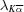= 1.542 Å for Cu and the K absorption edge λK = 1.488 (omitting units) of Ni but, unfortunately, leaves out the value of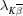for Cu. Figure 9.24 does not help; it refers to Mo not to Cu, which is not mentioned in the caption.

Chapter 10 is devoted to the diffraction of X-rays by polycrystalline materials. The Seeman–Bohlin camera, X-ray diffractometer (with Bragg–Brentano focusing geometry), Laue back reflection, Debye–Scherrer camera and texture goniometer are mentioned as diffraction techniques; lattice-parameter measurement, identification of unknown phases and the measurement of grain size and internal elastic strains as possible applications. A section is devoted to the measurement of fibre and sheet textures. The chapter ends with a discussion of the Rietveld method for structure refinement.

Chapter 11 deals with electron diffraction and its applications. Discussing the Ewald reflecting sphere construction for electron diffraction, precession electron diffraction (PED) and rotation electron diffraction (RED) are mentioned. Applications of electron diffraction to determine orientation relationships between adjacent crystals and to identify quasiperiodic crystals are described. The formation of Kikuchi patterns in transmission electron microscopy (TEM) and of electron backscattered diffraction (EBSD) patterns in scanning electron microscopy (SEM) are discussed.

In Chapter 12 the stereographic projection is introduced and the use of the Wulff net is described. The stereographic projection of symmetry elements is shown for some point groups. The stereographic projection is applied to represent preferred orientation between neighbouring crystal grains belonging to the same phase, and to orientation relationships between grains of different phases.

Chapter 13 introduces Fourier series of periodic functions and Fourier transforms of non-periodic ones. Fourier series are used to express the structure factors in terms of the charge distribution in a crystal and vice versa. New in the present edition is a section on methods of structural analysis discussing trial and error, Patterson maps, heavy-atom and isomorphous replacement techniques, direct methods and charge flipping. Finally, Fraunhofer diffraction patterns from a grating are analysed and Abbe's theory of image formation is illustrated.

Chapter 14 on physical properties of crystals and their description by tensors is new to the present edition. It starts by considering symmetric second-rank tensors and their representation quadrics for the case where the three eigenvalues are all positive, i.e. where the quadric is an ellipsoid. Then Neumann's principle is invoked for matter tensors to derive restrictions on the form and orientation of the ellipsoid. Thermal and electrical conductivity are given as examples where the representation quadric is an ellipsoid; thermal expansion is mentioned as an example where the representation quadric may be a hyperboloid in exceptional cases. Stress and strain are presented as symmetric second-rank field tensors, for which Neumann's principle does not apply. Optical properties of uniaxial crystals are discussed using the indicatrix. Piezoelectricity and elasticity are considered as examples of tensors of third and fourth rank, respectively. A number of mistakes have been noted. The left-hand side of the last equation in the section on piezoelectricity should be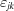instead of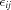. Changing from tensor to matrix notation in the section on elasticity, factors 2 are missing in the transition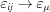and factors 2, 4 in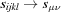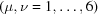. Other, less serious errors are: (temperature or electrical field gradient)' on p. 362 should be replaced by (temperature gradient or electrical field)'; in Fig. 14.9 the angles corresponding to OPD = 3λ/8 and λ/2 are given incorrectly; the anagram ceiiinosssttuv' on p. 380 stands for ut tensio, sic vis'.

Appendix 1 deals with computer programs, models and model building in crystallography; Appendix 2 lists and classifies polyhedra mentioned in the text. Appendix 3 is the longest with 46 pages. It gives biographical notes on crystallographers and scientists mentioned in the text, not always in strictly alphabetic order. Many of the 66 biographies deal at least as much with family background and education as with scientific achievements, especially in the case of British researchers. On p. 408 the predecessor of J. M. Bijvoet at the University of Utrecht, Ernst Cohen, is mentioned, who was killed by the Nazis in 1944. He had to retire in 1939 because he reached age 70, not because he was a Jew. Appendix 4 gives formulas for interplanar spacings, interplanar angles and volumes of unit cells, omitting the triclinic system. Relations between zones and planes are also collected. Appendix 5 introduces vectors and complex numbers for readers not familiar with these topics. Appendix 6 explains and lists systematic absences of diffraction peaks and discusses double diffraction. Appendix 7, which is new to the present edition, deals with group theory and its applications to crystallography. It contains a table of the 73 symmorphic space groups, where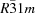and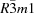should be replaced by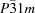and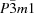, respectively.

There are other misprints in the text that I shall not mention individually. Some are caused by giving page numbers that have not been updated in the new edition.

The book presents crystallographic notions and diffraction methods in such a way that a reader confronted with a problem in materials science gets an indication of whether X-ray or electron diffraction might help to solve the problem. The text often refers to the section `Further reading', where books are recommended that can lead to a deeper understanding. However, the powerful applications of synchrotron X-ray radiation and of neutron sources are barely mentioned in the text.

The book contains many exercises; short answers are given to most of them, which makes the book also suitable for self-study.